# 1 Using Locator: Scenario and Examples

This chapter describes how to manage spatial (location-based) data, using the Oracle Locator feature of Oracle Database Express Edition (Oracle Database XE). It develops an extended example that shows the major steps required to store and use spatial data, and it includes some common queries.

It assumes that you are familiar with the main concepts and techniques described in Oracle Database 2 Day Developer's Guide, and especially that you know how to create SQL scripts and how to use SQL*Plus (in a SQL Developer worksheet or in a command-line window) to run SQL scripts.

This chapter includes the following major topics:

See Also:

## 1.1Overview of Spatial Data

Spatial data represents the essential location characteristics (typically longitude and latitude coordinates) of objects, which are also referred to as geometry objects and which include geometry types such as points, lines, and polygons. The location characteristics are stored using a special data type, SDO_GEOMETRY. Once spatial data is stored in an Oracle database, it can be easily manipulated, retrieved, and related to all other data stored in the database.

Oracle Locator, a feature of all editions of Oracle Database 10g, provides an integrated set of functions and procedures to efficiently store, manage, query. and analyze spatial data in an Oracle database, using standard SQL. Oracle Locator is a subset of Oracle Spatial and Graph, which is included in Oracle Database Enterprise Edition, and which adds high-end spatial functionality, including functions such as buffer generation, spatial aggregates, area calculations, and more; linear referencing; coordinate systems transformations; geocoding; a routing engine; topology and network data models; and support for georeferenced raster (GeoRaster) data.

See Also:

## 1.2 Scenario for Using Spatial Data

Consider the following business scenario. A company has several major retail stores. It needs to locate its customers who are near a given store, to inform them of new advertising promotions. To locate its customers and perform location-based analysis, the company must store location data for both its customers and stores.

The CUSTOMERS table has the following definition. (An actual customers table would have more information, but the definition is simplified for this scenario.)

```CREATE TABLE customers (
customer_id NUMBER,
last_name VARCHAR2(30),
first_name VARCHAR2(30),
street_address VARCHAR2(40),
city VARCHAR2(30),
state_province_code VARCHAR2(2),
postal_code VARCHAR2(9),
cust_geo_location SDO_GEOMETRY);
```

The STORES table has the following definition:

```CREATE TABLE stores (
store_id NUMBER,
description VARCHAR2(100),
street_address VARCHAR2(40),
city VARCHAR2(30),
state_province_code VARCHAR2(2),
postal_code VARCHAR2(9),
store_geo_location SDO_GEOMETRY);
```

Each table contains a column (cust_geo_location and store_geo_location) of the Oracle Spatial and Graph and Locator data type, SDO_GEOMETRY (described in Section 1.3, "Using the SDO_GEOMETRY Object Type"). In these tables, the SDO_GEOMETRY columns hold the geocoded location of each customer's residence and each store. These geocoded locations are stored as two-dimensional points, whose coordinates are the longitude and latitude values associated with the location. For example, longitude-latitude value pair of (-63.136, 52.4854) indicates the point at 63.136 degrees longitude west of the Greenwich prime meridian and 52.4854 degrees latitude north of the Equator.

Note:

Oracle Locator does not provide support for geocoding, that is, creating point geometry objects from specified address data. Oracle Spatial and Graph does support geocoding, using the SDO_GCDR package. However, if you already have the longitude and latitude points associated with your desired locations, you can use Oracle Locator to store and use the spatial data.

If you want, you can use the following statements in Example 1-1 to perform the operations in all examples associated with this customers and stores scenario. Many examples in the remaining sections use excerpts from these statements.

Example 1-1 SQL Script for Customers and Stores Scenario

```-- Clean up from any previous running of this procedure.

DROP TABLE customers;
DROP TABLE stores;
DROP INDEX customers_sidx;
DROP INDEX stores_sidx;
DELETE FROM USER_SDO_GEOM_METADATA
WHERE TABLE_NAME = 'CUSTOMERS' AND COLUMN_NAME = 'CUST_GEO_LOCATION';
DELETE FROM USER_SDO_GEOM_METADATA
WHERE TABLE_NAME = 'STORES' AND COLUMN_NAME = 'STORE_GEO_LOCATION';

-- Create table for customer information.

CREATE TABLE customers (
customer_id NUMBER,
last_name VARCHAR2(30),
first_name VARCHAR2(30),
street_address VARCHAR2(40),
city VARCHAR2(30),
state_province_code VARCHAR2(2),
postal_code VARCHAR2(9),
cust_geo_location SDO_GEOMETRY);

-- Create table for store information.

CREATE TABLE stores (
store_id NUMBER,
description VARCHAR2(100),
street_address VARCHAR2(40),
city VARCHAR2(30),
state_province_code VARCHAR2(2),
postal_code VARCHAR2(9),
store_geo_location SDO_GEOMETRY);

-- Insert customer data.

INSERT INTO customers VALUES
(1001,'Nichols', 'Alexandra',
'17 Maple Drive', 'Nashua', 'NH','03062',
SDO_GEOMETRY(2001, 8307,
SDO_POINT_TYPE (-71.48923,42.72347,NULL), NULL, NULL));

INSERT INTO customers VALUES
(1002,'Harris', 'Melvin',
'5543 Harrison Blvd', 'Reston', 'VA', '20190',
SDO_GEOMETRY(2001, 8307,
SDO_POINT_TYPE(-70.120133,44.795766,NULL), NULL, NULL));

INSERT INTO customers VALUES
(1003,'Chang', 'Marian',
'294 Main St', 'Concord', 'MA','01742',
SDO_GEOMETRY(2001, 8307,
SDO_POINT_TYPE (-71.351,42.4598,NULL), NULL, NULL));

INSERT INTO customers VALUES
(1004,'Williams', 'Thomas',
'84 Hayward Rd', 'Acton', 'MA','01720',
SDO_GEOMETRY(2001, 8307,
SDO_POINT_TYPE (-71.4559,42.4748,NULL), NULL, NULL));

INSERT INTO customers VALUES
(1005,'Rodriguez', 'Carla',
'9876 Pine Lane', 'Sudbury', 'MA','01776',
SDO_GEOMETRY(2001, 8307,
SDO_POINT_TYPE (-71.4242,42.3826,NULL), NULL, NULL));

INSERT INTO customers VALUES
(1006,'Adnani', 'Ramesh',
'1357 Appletree Ct', 'Falls Church', 'VA','22042 ',
SDO_GEOMETRY(2001, 8307,
SDO_POINT_TYPE (-77.1745,38.88505,NULL),NULL,NULL));

-- Insert stores data.

INSERT INTO stores VALUES
(101,'Nashua megastore',
'123 Commercial Way', 'Nashua', 'NH','03062',
SDO_GEOMETRY(2001, 8307,
SDO_POINT_TYPE (-71.49074,42.7229,NULL),NULL,NULL));

INSERT INTO stores VALUES
(102,'Reston store',
'99 Main Blvd', 'Reston', 'VA','22070',
SDO_GEOMETRY(2001, 8307,
SDO_POINT_TYPE (-77.34511,38.9521,NULL),NULL,NULL));

-- Add metadata to spatial view USER_SDO_GEOM_METADATA.

INSERT INTO USER_SDO_GEOM_METADATA (TABLE_NAME, COLUMN_NAME, DIMINFO, SRID)
VALUES ('CUSTOMERS', 'CUST_GEO_LOCATION',
SDO_DIM_ARRAY
(SDO_DIM_ELEMENT('LONG', -180.0, 180.0, 0.5),
SDO_DIM_ELEMENT('LAT', -90.0, 90.0, 0.5)),
8307);

INSERT INTO USER_SDO_GEOM_METADATA (TABLE_NAME, COLUMN_NAME, DIMINFO, SRID)
VALUES ('STORES', 'STORE_GEO_LOCATION',
SDO_DIM_ARRAY
(SDO_DIM_ELEMENT('LONG', -180.0, 180.0, 0.5),
SDO_DIM_ELEMENT('LAT', -90.0, 90.0, 0.5)),
8307);

-- Create spatial indexes.

CREATE INDEX customers_sidx ON customers(cust_geo_location)
INDEXTYPE IS mdsys.spatial_index;

CREATE INDEX stores_sidx ON stores(store_geo_location)
INDEXTYPE IS mdsys.spatial_index;

-- Perform location-based queries.

-- Find the 3 closest customers to store_id = 101.

SELECT /*+ordered*/
c.customer_id,
c.first_name,
c.last_name
FROM stores s,
customers c
WHERE s.store_id = 101
AND sdo_nn (c.cust_geo_location, s.store_geo_location, 'sdo_num_res=3')
= 'TRUE';

-- Find the 3 closest customers to store_id = 101, and
-- order the results by distance.

SELECT /*+ordered index(c customers_sidx) */
c.customer_id,
c.first_name,
c.last_name,
sdo_nn_distance (1) distance
FROM stores s,
customers c
WHERE s.store_id = 101
AND sdo_nn
(c.cust_geo_location, s.store_geo_location, 'sdo_num_res=3', 1)
= 'TRUE'
ORDER BY distance;

-- Find all the customers within 100 miles of store_id = 101

SELECT /*+ordered*/
c.customer_id,
c.first_name,
c.last_name
FROM stores s,
customers c
WHERE s.store_id = 101
AND sdo_within_distance (c.cust_geo_location,
s.store_geo_location,
'distance = 100 unit=MILE') = 'TRUE';
```

## 1.3 Using the SDO_GEOMETRY Object Type

This section introduces the SDO_GEOMETRY type, which you must use to store spatial data as geometry objects. It does not contain a detailed explanation, which is provided in Oracle Spatial and Graph Developer's Guide.

The geometric description of a spatial object is stored in a single row, in a single column of object type SDO_GEOMETRY in a user-defined table. Any table that has a column of type SDO_GEOMETRY must have another column, or set of columns, that defines a unique primary key for that table. The SDO_GEOMETRY type is defined as:

```CREATE TYPE sdo_geometry AS OBJECT (
SDO_GTYPE NUMBER,
SDO_SRID NUMBER,
SDO_POINT SDO_POINT_TYPE,
SDO_ELEM_INFO SDO_ELEM_INFO_ARRAY,
SDO_ORDINATES SDO_ORDINATE_ARRAY);
```

The SDO_GTYPE attribute indicates the type of the geometry. The SDO_GTYPE value is 4 digits in the format dltt, where d identifies the number of dimensions (2, 3, or 4), l identifies the linear referencing measure dimension for a three-dimensional linear referencing system (LRS) geometry, and tt identifies the geometry type (00 through 07). (LRS geometries are not supported with Oracle Locator, so the l value must be 0.) Examples of SDO_GTYPE values include 2001 for a two-dimensional point, 2002 for a two-dimensional line string, and 2003 for a two-dimensional polygon.

The SDO_SRID attribute can be used to identify a coordinate system (spatial reference system) to be associated with the geometry. If SDO_SRID is null, no coordinate system is associated with the geometry. If SDO_SRID is not null, it must contain a value from the SRID column of the SDO_COORD_REF_SYS table, and this value must be inserted into the SRID column of the USER_SDO_GEOM_METADATA view. The SRID value 8307 is associated with the widely used WGS84 longitude/latitude coordinate system.

The SDO_POINT attribute is used only for point data, and it is defined using the SDO_POINT_TYPE object type, which has the attributes X, Y, and Z, all of type NUMBER. If the SDO_ELEM_INFO and SDO_ORDINATES arrays are both null, and the SDO_POINT attribute is non-null, then the X and Y values are considered to be the coordinates for a point geometry. Otherwise, the SDO_POINT attribute is ignored. You should store point geometries in the SDO_POINT attribute for optimal storage.

The SDO_ELEM_INFO attribute is defined using a varying length array of numbers. This attribute lets you know how to interpret the ordinates stored in the SDO_ORDINATES attribute. Each triplet set of numbers is interpreted as follows:

• SDO_STARTING_OFFSET -- Indicates the offset within the SDO_ORDINATES array where the first ordinate for this element is stored. Offset values start at 1 and not at 0. Thus, the first ordinate for the first element will be at SDO_GEOMETRY.SDO_ORDINATES(1). If there is a second element, its first ordinate will be at SDO_GEOMETRY.SDO_ORDINATES(n), where n reflects the position within the SDO_ORDINATE_ARRAY definition (for example, 19 for the 19th number).

• SDO_ETYPE -- Indicates the type of the element.

• SDO_INTERPRETATION -- Means one of two things, depending on whether or not SDO_ETYPE is a compound element. If the SDO_ETYPE is not a compound element (1, 2, 1003, or 2003), the interpretation attribute determines how the sequence of ordinates for this element is interpreted. For example, a line string or polygon boundary may be made up of a sequence of connected straight line segments or circular arcs. If SDO_ETYPE is a compound element (4, 1005, or 2005), this field specifies how many subsequent triplet values are part of the element.

The valid SDO_ETYPE and SDO_INTERPRETATION value pairs are described in detail in Oracle Spatial and Graph Developer's Guide.

The SDO_ORDINATES attribute is defined using a varying length array (1048576) of NUMBER type that stores the coordinate values that make up the boundary of a spatial object. This array must always be used in conjunction with the SDO_ELEM_INFO varying length array. The values in the array are ordered by dimension. For example, a polygon whose boundary has four two-dimensional points is stored as {X1, Y1, X2, Y2, X3, Y3, X4, Y4, X1, Y1}. The number of dimensions associated with each point is stored as metadata in the USER_SDO_GEOM_METADATA view.

The SDO_GEOMETRY constructor is used to create a geometry object. The examples in Section 1.9, "Using Non-Point Geometry Types" show the use of the SDO_GEOMETRY constructor to create many different kinds of geometry objects.

## 1.4Loading Customer and Store Information, Including Locations

You will use transactional insert operations to add new customers and their locations to the CUSTOMERS table, and new stores and their locations into the STORES table. A location can be stored as a point in an SDO_GEOMETRY column in a table. The customer or store location is associated with longitude and latitude values on the Earth's surface (for example, -63.136, 52.4854). Oracle Locator requires that you place the longitude value before the latitude value.

Example 1-2 inserts some rows into the CUSTOMERS and STORES tables. In the INSERT statements, the SDO_GEOMETRY constructor is used to insert the point location.

Example 1-2 Inserting Customer and Store Records

```-- Insert customer data.

INSERT INTO customers VALUES
(1001,'Nichols', 'Alexandra',
'17 Maple Drive', 'Nashua', 'NH','03062',
SDO_GEOMETRY(2001, 8307,
SDO_POINT_TYPE (-71.48923,42.72347,NULL), NULL, NULL));

INSERT INTO customers VALUES
(1002,'Harris', 'Melvin',
'5543 Harrison Blvd', 'Reston', 'VA', '20190',
SDO_GEOMETRY(2001, 8307,
SDO_POINT_TYPE(-70.120133,44.795766,NULL), NULL, NULL));

INSERT INTO customers VALUES
(1003,'Chang', 'Marian',
'294 Main St', 'Concord', 'MA','01742',
SDO_GEOMETRY(2001, 8307,
SDO_POINT_TYPE (-71.351,42.4598,NULL), NULL, NULL));

INSERT INTO customers VALUES
(1004,'Williams', 'Thomas',
'84 Hayward Rd', 'Acton', 'MA','01720',
SDO_GEOMETRY(2001, 8307,
SDO_POINT_TYPE (-71.4559,42.4748,NULL), NULL, NULL));

INSERT INTO customers VALUES
(1005,'Rodriguez', 'Carla',
'9876 Pine Lane', 'Sudbury', 'MA','01776',
SDO_GEOMETRY(2001, 8307,
SDO_POINT_TYPE (-71.4242,42.3826,NULL), NULL, NULL));

INSERT INTO customers VALUES
(1006,'Adnani', 'Ramesh',
'1357 Appletree Ct', 'Falls Church', 'VA','22042 ',
SDO_GEOMETRY(2001, 8307,
SDO_POINT_TYPE (-77.1745,38.88505,NULL),NULL,NULL));

-- Insert stores data.

INSERT INTO stores VALUES
(101,'Nashua megastore',
'123 Commercial Way', 'Nashua', 'NH','03062',
SDO_GEOMETRY(2001, 8307,
SDO_POINT_TYPE (-71.49074, 42.7229,NULL),NULL,NULL));

INSERT INTO stores VALUES
(102,'Reston store',
'99 Main Blvd', 'Reston', 'VA','22070',
SDO_GEOMETRY(2001, 8307,
SDO_POINT_TYPE (-77.34511, 38.9521,NULL),NULL,NULL));
```

## 1.5Updating the Spatial Metadata

For each spatial column (type SDO_GEOMETRY), you must insert an appropriate row into the USER_SDO_GEOM_METADATA view to reflect the dimensional information for the area in which the data is located. You must do this before creating spatial indexes (see Section 1.6, "Creating Spatial Indexes") on the spatial columns.

The USER_SDO_GEOM_METADATA view has the following definition:

```(
TABLE_NAME   VARCHAR2(32),
COLUMN_NAME  VARCHAR2(32),
DIMINFO      SDO_DIM_ARRAY,
SRID         NUMBER
);
```

The DIMINFO column is a varying length array of an object type, ordered by dimension, and has one entry for each dimension. The SDO_DIM_ARRAY type is defined as follows:

```Create Type SDO_DIM_ARRAY as VARRAY(4) of SDO_DIM_ELEMENT;
```

The SDO_DIM_ELEMENT type is defined as:

```Create Type SDO_DIM_ELEMENT as OBJECT (
SDO_DIMNAME VARCHAR2(64),
SDO_LB NUMBER,
SDO_UB NUMBER,
SDO_TOLERANCE NUMBER);
```

The SDO_DIM_ARRAY instance is of size n if there are n dimensions. That is, DIMINFO contains 2 SDO_DIM_ELEMENT instances for two-dimensional geometries, 3 instances for three-dimensional geometries, and 4 instances for four-dimensional geometries. Each SDO_DIM_ELEMENT instance in the array must have valid (not null) values for the SDO_LB (lower bound), SDO_UB (upper bound), and SDO_TOLERANCE (tolerance) attributes.

Tolerance reflects the distance that two points can be apart and still be considered the same (for example, to accommodate rounding errors), and thus reflects the precision of the spatial data. The tolerance value must be a positive number greater than zero.

Example 1-3 inserts rows into the USER_SDO_GEOM_METADATA view, with dimensional information for each spatial column. In both cases, the dimensional range is the entire Earth, and the coordinate system is the widely used WGS84 (longitude/latitude) system (spatial reference ID = 8307).

Example 1-3 Updating the Spatial Metadata

```INSERT INTO USER_SDO_GEOM_METADATA (TABLE_NAME, COLUMN_NAME, DIMINFO, SRID)
VALUES ('CUSTOMERS', 'CUST_GEO_LOCATION',
SDO_DIM_ARRAY
(SDO_DIM_ELEMENT('LONG', -180.0, 180.0, 0.5),
SDO_DIM_ELEMENT('LAT', -90.0, 90.0, 0.5)),
8307);

INSERT INTO USER_SDO_GEOM_METADATA (TABLE_NAME, COLUMN_NAME, DIMINFO, SRID)
VALUES ('STORES', 'STORE_GEO_LOCATION',
SDO_DIM_ARRAY
(SDO_DIM_ELEMENT('LONG', -180.0, 180.0, 0.5),
SDO_DIM_ELEMENT('LAT', -90.0, 90.0, 0.5)),
8307);
```

In Example 1-3, the longitude dimension of -180.0,180.0 and latitude dimension of -90.90 are required for geodetic data using the WGS84 coordinate system. The tolerance value of 0.5 means that any points less than one-half meter apart are considered to be the same point by any location-based operators or functions.

## 1.6Creating Spatial Indexes

Spatial indexes are required for many queries that use Locator operators, and are important for performance for most spatial queries. Before you use spatial data for analysis or queries, create a spatial index on each spatial column. To create a spatial index, use the CREATE INDEX statement, and specify the INDEXTYPE IS MDSYS.SPATIAL_INDEX clause.

To create a spatial index, the database user must have the CREATE TABLE privilege.

Example 1-4 creates spatial indexes on the CUSTOMERS.CUST_GEO_LOCATION and STORES.STORE_GEO_LOCATION columns.

Example 1-4 Creating the Spatial Indexes

```CREATE INDEX customers_sidx ON customers(cust_geo_location)
INDEXTYPE IS mdsys.spatial_index;

CREATE INDEX stores_sidx ON stores(store_geo_location)
INDEXTYPE IS mdsys.spatial_index;
```

## 1.7Querying Spatial Data

After you have created and populated spatial tables, updated the spatial metadata, and created spatial indexes, you can use Oracle Locator operator and functions to perform location-based queries. This section shows some queries to find the closest customers to a store and all customers within a specified distance of a store.

Example 1-5 shows the SQL statement and the output for finding the three closest customers to the store with the STORE_ID value of 101. This example uses the SDO_NN ("nearest neighbors") operator.

Example 1-5 Finding Closest Customers to a Store

```SELECT /*+ordered*/
c.customer_id,
c.first_name,
c.last_name
FROM stores s,
customers c
WHERE s.store_id = 101
AND sdo_nn (c.cust_geo_location, s.store_geo_location, 'sdo_num_res=3') = 'TRUE';

CUSTOMER_ID FIRST_NAME                     LAST_NAME
----------- ------------------------------ ------------------------------
1001 Alexandra                      Nichols
1003 Marian                         Chang
1004 Thomas                         Williams
```

In Example 1-5:

• The `/*+ordered*/` hint is a hint to the optimizer, which ensures that the STORES table is searched first.

• The SDO_NN operator returns the SDO_NUM_RES value of the customers from the CUSTOMERS table who are closest to store 101. The first argument to SDO_NN (c.cust_geo_location in the example) is the column to search. The second argument to SDO_NN (s.storeh_geo_location in the example) is the location you want to find the neighbors nearest to. No assumptions should be made about the order of the returned results. For example, the first row returned is not guaranteed to be the customer closest to store 101. If two or more customers are an equal distance from the store, either of the customers may be returned on subsequent calls to SDO_NN.

• When you use the SDO_NUM_RES parameter, no other constraints are used in the WHERE clause. SDO_NUM_RES takes only proximity into account. For example, if you added a criterion to the WHERE clause because you wanted the five closest customers that resided in NY, and four of the five closest customers resided in NJ, the preceding query would return one row. This behavior is specific to the SDO_NUM_RES parameter, and its results may not be what you are looking for.

Example 1-6 extends Example 1-5 by showing the SQL statement and the output (reformatted for readability) for finding the three closest customers to the store with the STORE_ID value of 101, and ordering the results by distance (in meters) from the store. This example uses the SDO_NN_DISTANCE ancillary operator.

Example 1-6 Finding Closest Customers, Ordered by Distance from Store

```SELECT /*+ordered index(c customers_sidx) */
c.customer_id,
c.first_name,
c.last_name,
sdo_nn_distance (1) distance
FROM stores s,
customers c
WHERE s.store_id = 101
AND sdo_nn
(c.cust_geo_location, s.store_geo_location, 'sdo_num_res=3', 1) = 'TRUE'
ORDER BY distance;

CUSTOMER_ID FIRST_NAME  LAST_NAME   DISTANCE
----------- ----------- ----------  ------------------
1001 Alexandra   Nichols     138.94486

1004 Thomas      Williams    27708.0946

1003 Marian      Chang       31396.4521
```

In Example 1-6:

• The `index(c customers_sidx)` hint is required here to force the spatial index on the CUSTOMERS table to be used, because of a problem in this release with spatial queries that use an `ORDER BY` clause.

• The SDO_NN_DISTANCE operator is an ancillary operator to the SDO_NN operator; it can only be used within the SDO_NN operator. The argument for this operator is a number that matches the number specified as the last argument of SDO_NN; in this example it is 1. There is no hidden meaning to this argument; it is simply a tag. With SDO_NN_DISTANCE, you can order the results by distance and guarantee that the first row returned is the closest. If the data you are querying is stored as longitude and latitude, the default unit for SDO_NN_DISTANCE is meters.

• The SDO_NN operator also has a `unit` parameter that determines the unit of measurement returned by SDO_NN_DISTANCE; however, it is not used in this example.

• The `ORDER BY distance` clause ensures that the distances are returned in order, with the shortest distance first.

Example 1-7 shows the SQL statement and the output for finding all customers within 100 miles of the store with the STORE_ID value of 101. This example uses the SDO_WITHIN_DISTANCE operator.

Example 1-7 Finding Customers Within 100 Miles of a Store

```SELECT /*+ordered*/
c.customer_id,
c.first_name,
c.last_name
FROM stores s,
customers c
WHERE s.store_id = 101
AND sdo_within_distance (c.cust_geo_location,
s.store_geo_location,
'distance = 100 unit=MILE') = 'TRUE';

CUSTOMER_ID FIRST_NAME                     LAST_NAME
----------- ------------------------------ ------------------------------
1005 Carla                          Rodriguez
1004 Thomas                         Williams
1003 Marian                         Chang
1001 Alexandra                      Nichols
```

In Example 1-7:

• The SDO_WITHIN_DISTANCE operator returns the customers from the customers table that are within 100 miles of store 101. The first parameter to SDO_WITHIN_DISTANCE (`c.cust_geo_location` in the example) is the column to search. The second parameter (`s.store_geo_location` in the example) is the location from which you want to determine the distances. No assumptions should be made about the order of the returned results. For example, the first row returned is not guaranteed to be the customer closest to store 101.

• The `distance` keyword specifies the distance value (100 in this example).

• The `unit` keyword specifies the unit of measure for the `distance` keyword. The default unit is the unit of measure associated with the data. For longitude and latitude data, the default is meters; however, in this example it is miles.

## 1.8 Running the Scenario Script

You can create a SQL script that includes the operations described in preceding sections about the scenario for using spatial data: creating the tables, loading data into the tables, updating the spatial metadata, creating the spatial indexes, and querying the spatial data. For example, you could create a script containing the statements in Example 1-1 to perform these operations for the sample scenario.

After you create the SQL script, you can test it by running it.

Note:

You cannot use the Express Edition SQL Commands or SQL Scripts features to run a SQL script or enter a SQL statement that creates or requires a spatial index. You must instead use SQL*Plus, such as by selecting Run SQL Command Line from the Express Edition menus, and connect as a database user that has the Create Table privilege.

Follow these steps to run the SQL script:

1. On the Express Edition menus, select Run SQL Command Line. Specifically:

• On Windows, from the Start menu, select Programs (or All Programs), then Oracle Database 10g Express Edition, then Run SQL Command Line.

• On Linux, click the Application menu (on Gnome) or the K menu (on KDE), then point to Oracle Database 10g Express Edition, then Run SQL Command Line.

2. In the Run SQL Command Line window, connect to the Express Edition database as the user in whose schema you want to execute the SQL script. The following example connects as user `smith` with the password `jane`:

```SQL> connect smith/jane
```
3. Use the SQL*Plus @ ("at" sign) or @@ (double "at" sign) command to specify the path and file name of the SQL script file. The following example (showing Windows syntax) runs a SQL script file named `locator_scenario.sql`:

```SQL> @c:\my_scripts\locator_scenario
```

If you do not specify a file extension, `.sql` is assumed.

Figure 1-1 shows the results of one of the queries in the scenario script shown in Example 1-1. This query returns the three customers nearest to the store that has store ID 101, with the distance in meters of each customer's home from the store, and with the results ordered by distance from the store.

Figure 1-1 Location Query and Results: Three Closest Customers, with Distance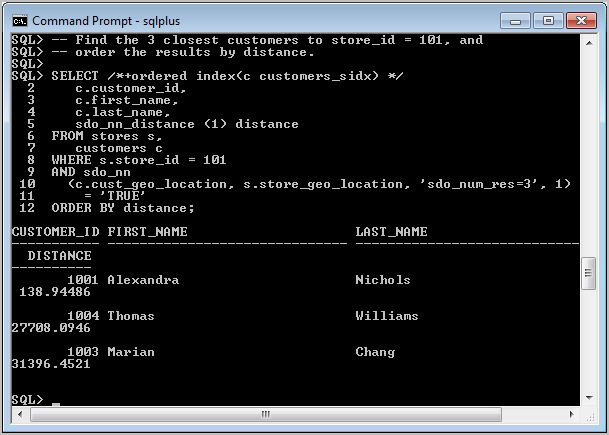Description of "Figure 1-1 Location Query and Results: Three Closest Customers, with Distance"

As shown in Figure 1-1, the results indicate that Alexandra Nichols lives about 139 meters from the store, Thomas Williams lives about 27.7 kilometers from the store, and Marian Chang lives about 31.4 kilometers from the store.

## 1.9 Using Non-Point Geometry Types

The examples so far in this chapter have shown point geometries, because the scenario involved the use of addresses that are represented as points. However, with Locator you can store and use many other types of geometries, such as lines (representing rivers, roads, pipelines, and so on) and polygons (representing any areas, such as counties, states, provinces, countries, and so on).

This section contains examples of creating non-point geometries. The examples include some explanation, but for detailed explanations of these geometry types, see Oracle Spatial and Graph Developer's Guide.

The examples do not use the WGS84 longitude/latitude coordinate system. Instead, they use a null coordinate system, which is not Earth-based and which reflects an arbitrary grid on a plane. All examples except those in Section 1.9.7, "Several Geometry Types" use a table named COLA_MARKETS. The following statements were used to create the COLA_MARKETS table, add its metadata to the USER_SDO_GEOMETRY view, and create a spatial index for its SDO_GEOMETRY column.

```-- Create a table for cola (soft drink) markets in a
-- given geography (such as city or state).
-- Each row will be an area of interest for a specific
-- cola (for example, where the cola is most preferred
-- by residents, where the manufacturer believes the
-- cola has growth potential, and so on).

CREATE TABLE cola_markets (
mkt_id NUMBER PRIMARY KEY,
name VARCHAR2(32),
shape SDO_GEOMETRY);

---------------------------------------------------------------------------
-- UPDATE METADATA VIEW --
---------------------------------------------------------------------------
-- Update the USER_SDO_GEOM_METADATA view. This is required
-- before the Spatial index can be created. Do this only once for each
-- layer (that is, table-column combination; here: COLA_MARKETS and SHAPE).

INSERT INTO user_sdo_geom_metadata
(TABLE_NAME,
COLUMN_NAME,
DIMINFO,
SRID)
VALUES (
'cola_markets',
'shape',
SDO_DIM_ARRAY(   -- 20X20 grid
SDO_DIM_ELEMENT('X', 0, 20, 0.005),
SDO_DIM_ELEMENT('Y', 0, 20, 0.005)
),
NULL   -- SRID
);

-------------------------------------------------------------------
-- CREATE THE SPATIAL INDEX --
-------------------------------------------------------------------
CREATE INDEX cola_spatial_idx
ON cola_markets(shape)
INDEXTYPE IS MDSYS.SPATIAL_INDEX;
```

This section covers the following geometry types:

### 1.9.1Polygon

Figure 1-2 illustrates a polygon.

Figure 1-2 Polygon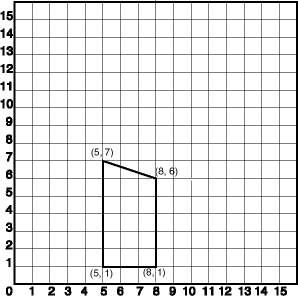Description of "Figure 1-2 Polygon"

In the SDO_GEOMETRY definition of the geometry illustrated in Figure 1-2:

• SDO_GTYPE = 2003. The 2 indicates two-dimensional, and the 3 indicates a polygon.

• SDO_SRID = NULL.

• SDO_POINT = NULL.

• SDO_ELEM_INFO = (1,1003,1). The 1 in 1003 indicates an exterior polygon ring. The final 1 in 1,1003,1 indicates that this is a simple polygon whose vertices are connected by straight line segments, and you must specify coordinates for each vertex point, with the last set of coordinates being the same as the first set.

• SDO_ORDINATES = (5,1, 8,1, 8,6, 5,7, 5,1). These identify the vertices of the polygon, with the first and last coordinates the same. Because this is an exterior polygon ring (this simple pylon has no interior ring), the coordinates are in counterclockwise order.

Example 1-8 shows a SQL statement that inserts the geometry illustrated in Figure 1-2 into the database.

Example 1-8 SQL Statement to Insert a Polygon

```INSERT INTO cola_markets VALUES(
301,
'polygon',
SDO_GEOMETRY(
2003,  -- two-dimensional polygon
NULL,
NULL,
SDO_ELEM_INFO_ARRAY(1,1003,1), -- one polygon (exterior polygon ring)
SDO_ORDINATE_ARRAY(5,1, 8,1, 8,6, 5,7, 5,1)
)
);
```

### 1.9.2Rectangle

Figure 1-3 illustrates a rectangle.

Figure 1-3 Rectangle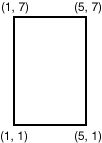Description of "Figure 1-3 Rectangle"

In the SDO_GEOMETRY definition of the geometry illustrated in Figure 1-3:

• SDO_GTYPE = 2003. The 2 indicates two-dimensional, and the 3 indicates a polygon.

• SDO_SRID = NULL.

• SDO_POINT = NULL.

• SDO_ELEM_INFO = (1, 1003, 3). The final 3 in 1,1003,3 indicates that this is a rectangle. Because it is a rectangle, only two ordinates are specified in SDO_ORDINATES (lower-left and upper-right).

• SDO_ORDINATES = (1,1, 5,7). These identify the lower-left and upper-right ordinates of the rectangle.

Example 1-9 shows a SQL statement that inserts the geometry illustrated in Figure 1-3 into the database.

Example 1-9 SQL Statement to Insert a Rectangle

```INSERT INTO cola_markets VALUES(
302,
'rectangle',
SDO_GEOMETRY(
2003,  -- two-dimensional polygon
NULL,
NULL,
SDO_ELEM_INFO_ARRAY(1,1003,3), -- one rectangle (1003 = exterior)
SDO_ORDINATE_ARRAY(1,1, 5,7) -- only 2 points needed to
-- define rectangle (lower left and upper right) with
-- Cartesian-coordinate data
)
);
```

### 1.9.3Polygon with a Hole

Figure 1-4 illustrates a polygon consisting of two elements: an exterior polygon ring and an interior polygon ring. The inner element in this example is treated as a void (a hole).

Figure 1-4 Polygon with a Hole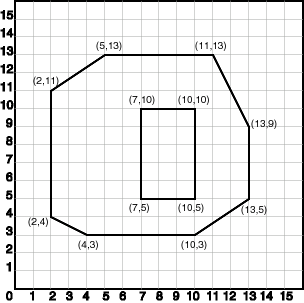Description of "Figure 1-4 Polygon with a Hole"

In the SDO_GEOMETRY definition of the geometry illustrated in Figure 1-4:

• SDO_GTYPE = 2003. The 2 indicates two-dimensional, and the 3 indicates a polygon.

• SDO_SRID = NULL.

• SDO_POINT = NULL.

• SDO_ELEM_INFO = (1,1003,1, 19,2003,1). There are two triplet elements: 1,1003,1 and 19,2003,1.

1003 indicates that the element is an exterior polygon ring; 2003 indicates that the element is an interior polygon ring.

19 indicates that the second element (the interior polygon ring) ordinate specification starts at the 19th number in the SDO_ORDINATES array (that is, 7, meaning that the first point is 7,5).

• SDO_ORDINATES = (2,4, 4,3, 10,3, 13,5, 13,9, 11,13, 5,13, 2,11, 2,4, 7,5, 7,10, 10,10, 10,5, 7,5).

• The area (SDO_GEOM.AREA function) of the polygon is the area of the exterior polygon minus the area of the interior polygon. In this example, the area is 84 (99 - 15).

• The perimeter (SDO_GEOM.LENGTH function) of the polygon is the perimeter of the exterior polygon plus the perimeter of the interior polygon. In this example, the perimeter is 52.9193065 (36.9193065 + 16).

Example 1-10 shows a SQL statement that inserts the geometry illustrated in Figure 1-4 into the database.

Example 1-10 SQL Statement to Insert a Polygon with a Hole

```INSERT INTO cola_markets VALUES(
303,
'polygon_with_hole',
SDO_GEOMETRY(
2003,  -- two-dimensional polygon
NULL,
NULL,
SDO_ELEM_INFO_ARRAY(1,1003,1, 19,2003,1), -- polygon with hole
SDO_ORDINATE_ARRAY(2,4, 4,3, 10,3, 13,5, 13,9, 11,13, 5,13, 2,11, 2,4,
7,5, 7,10, 10,10, 10,5, 7,5)
)
);
```

An example of such a "polygon with a hole" might be a land mass (such as a country or an island) with a lake inside it. Of course, an actual land mass might have many such interior polygons: each one would require a triplet element in SDO_ELEM_INFO, plus the necessary ordinate specification.

Exterior and interior rings cannot be nested. For example, if a country has a lake and there is an island in the lake (and perhaps a lake on the island), a separate polygon must be defined for the island; the island cannot be defined as an interior polygon ring within the interior polygon ring of the lake.

In a multipolygon (polygon collection), rings must be grouped by polygon, and the first ring of each polygon must be the exterior ring. For example, consider a polygon collection that contains two polygons (A and B):

• Polygon A (one interior "hole"): exterior ring A0, interior ring A1

• Polygon B (two interior "holes"): exterior ring B0, interior ring B1, interior ring B2

The elements in SDO_ELEM_INFO and SDO_ORDINATES must be in one of the following orders (depending on whether you specify Polygon A or Polygon B first):

• A0, A1; B0, B1, B2

• B0, B1, B2; A0, A1

### 1.9.4Line String

Figure 1-5 illustrates a line string made up of three straight line segments, starting at (3,2). Four points are required to represent this shape: (3,2), (4,6), (6,4), and (14,7).

Figure 1-5 Line String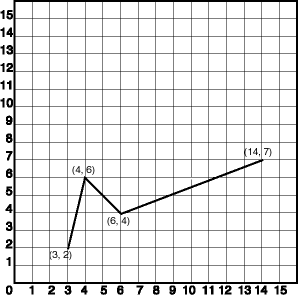Description of "Figure 1-5 Line String"

In the SDO_GEOMETRY definition of the geometry illustrated in Figure 1-5:

• SDO_GTYPE = 2002. The first 2 indicates two-dimensional, and the second 2 indicates one or more line segments.

• SDO_SRID = NULL.

• SDO_POINT = NULL.

• SDO_ELEM_INFO = (1,2,1). The 2,1 in 1,2,1 indicates a line string whose vertices are connected by straight line segments.

• SDO_ORDINATES = (3,2, 4,6, 6,4, 14,7).

Example 1-11 shows a SQL statement that inserts the geometry illustrated in Figure 1-5 into the database.

Example 1-11 SQL Statement to Insert a Line String

```INSERT INTO cola_markets VALUES(
304,
'line_string',
SDO_GEOMETRY(
2002,
NULL,
NULL,
SDO_ELEM_INFO_ARRAY(1,2,1), -- line string
SDO_ORDINATE_ARRAY(3,2, 4,6, 6,4, 14,7)
)
);
```

### 1.9.5Compound Line String

Figure 1-6 illustrates a crescent-shaped object represented as a compound line string made up of one straight line segment and one circular arc. Four points are required to represent this shape: points (10,10) and (10,14) describe the straight line segment, and points (10,14), (6,10), and (14,10) describe the circular arc.

Figure 1-6 Compound Line String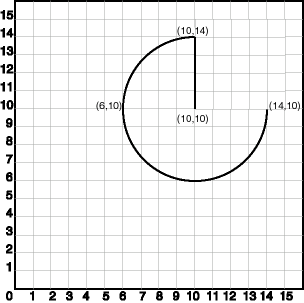Description of "Figure 1-6 Compound Line String"

In the SDO_GEOMETRY definition of the geometry illustrated in Figure 1-6:

• SDO_GTYPE = 2002. The first 2 indicates two-dimensional, and the second 2 indicates one or more line segments.

• SDO_SRID = NULL.

• SDO_POINT = NULL.

• SDO_ELEM_INFO = (1,4,2, 1,2,1, 3,2,2). There are three triplet elements: 1,4,2, 1,2,1, and 3,2,2.

The first triplet indicates that this element is a compound line string made up of two subelement line strings, which are described with the next two triplets.

The second triplet indicates that the line string is made up of straight line segments and that the ordinates for this line string start at offset 1. The end point of this line string is determined by the starting offset of the second line string, 3 in this instance.

The third triplet indicates that the second line string is made up of circular arcs with ordinates starting at offset 3. The end point of this line string is determined by the starting offset of the next element or the current length of the SDO_ORDINATES array, if this is the last element.

• SDO_ORDINATES = (10,10, 10,14, 6,10, 14,10).

Example 1-12 shows a SQL statement that inserts the geometry illustrated in Figure 1-6 into the database.

Example 1-12 SQL Statement to Insert a Compound Line String

```INSERT INTO cola_markets VALUES(
305,
'compound_line_string',
SDO_GEOMETRY(
2002,
NULL,
NULL,
SDO_ELEM_INFO_ARRAY(1,4,2, 1,2,1, 3,2,2), -- compound line string
SDO_ORDINATE_ARRAY(10,10, 10,14, 6,10, 14,10)
)
);
```

### 1.9.6Compound Polygon

Figure 1-7 illustrates an ice cream cone-shaped object represented as a compound polygon made up of one straight line segment and one circular arc. Five points are required to represent this shape: points (6,10), (10,1), and (14,10) describe one acute angle-shaped line string, and points (14,10), (10,14), and (6,10) describe the circular arc. The starting point of the line string and the ending point of the circular arc are the same point (6,10). The SDO_ELEM_INFO array contains three triplets for this compound line string. These triplets are {(1,1005,2), (1,2,1), (5,2,2)}.

Figure 1-7 Compound Polygon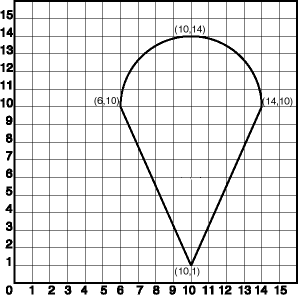Description of "Figure 1-7 Compound Polygon"

In the SDO_GEOMETRY definition of the geometry illustrated in Figure 1-7:

• SDO_GTYPE = 2003. The 2 indicates two-dimensional, and the 3 indicates a polygon.

• SDO_SRID = NULL.

• SDO_POINT = NULL.

• SDO_ELEM_INFO = (1,1005,2, 1,2,1, 5,2,2). There are three triplet elements: 1,1005,2, 1,2,1, and 5,2,2.

The first triplet indicates that this element is a compound polygon made up of two subelement line strings, which are described using the next two triplets.

The second triplet indicates that the first subelement line string is made up of straight line segments and that the ordinates for this line string start at offset 1. The end point of this line string is determined by the starting offset of the second line string, 5 in this instance. Because the vertices are two-dimensional, the coordinates for the end point of the first line string are at ordinates 5 and 6.

The third triplet indicates that the second subelement line string is made up of a circular arc with ordinates starting at offset 5. The end point of this line string is determined by the starting offset of the next element or the current length of the SDO_ORDINATES array, if this is the last element.

• SDO_ORDINATES = (6,10, 10,1, 14,10, 10,14, 6,10).

Example 1-13 shows a SQL statement that inserts the geometry illustrated in Figure 1-7 into the database.

Example 1-13 SQL Statement to Insert a Compound Polygon

```INSERT INTO cola_markets VALUES(
306,
'compound_polygon',
SDO_GEOMETRY(
2003,  -- two-dimensional polygon
NULL,
NULL,
SDO_ELEM_INFO_ARRAY(1,1005,2, 1,2,1, 5,2,2), -- compound polygon
SDO_ORDINATE_ARRAY(6,10, 10,1, 14,10, 10,14, 6,10)
)
);
```

### 1.9.7 Several Geometry Types

Example 1-14 creates a table and inserts various geometries, including multipoints (point clusters), multipolygons, and collections. At the end, it calls the SDO_GEOM.VALIDATE_GEOMETRY_WITH_CONTEXT function to validate the inserted geometries. Note that some geometries are deliberately invalid, and their descriptions include the string `INVALID`.

Example 1-14 SQL Statements to Insert Various Geometries

```CREATE TABLE t1 (
i NUMBER,
d VARCHAR2(50),
g SDO_GEOMETRY
);
INSERT INTO t1 (i, d, g)
VALUES (
1,
'Line segment',
sdo_geometry (2002, null, null, sdo_elem_info_array (1,2,1),
sdo_ordinate_array (10,10, 20,10))
);
INSERT INTO t1 (i, d, g)
VALUES (
2,
'Arc segment',
sdo_geometry (2002, null, null, sdo_elem_info_array (1,2,2),
sdo_ordinate_array (10,15, 15,20, 20,15))
);
INSERT INTO t1 (i, d, g)
VALUES (
3,
'Line string',
sdo_geometry (2002, null, null, sdo_elem_info_array (1,2,1),
sdo_ordinate_array (10,25, 20,30, 25,25, 30,30))
);
INSERT INTO t1 (i, d, g)
VALUES (
4,
'Arc string',
sdo_geometry (2002, null, null, sdo_elem_info_array (1,2,2),
sdo_ordinate_array (10,35, 15,40, 20,35, 25,30, 30,35))
);
INSERT INTO t1 (i, d, g)
VALUES (
5,
'Compound line string',
sdo_geometry (2002, null, null,
sdo_elem_info_array (1,4,3, 1,2,1, 3,2,2, 7,2,1),
sdo_ordinate_array (10,45, 20,45, 23,48, 20,51, 10,51))
);
INSERT INTO t1 (i, d, g)
VALUES (
6,
'Closed line string',
sdo_geometry (2002, null, null, sdo_elem_info_array (1,2,1),
sdo_ordinate_array (10,55, 15,55, 20,60, 10,60, 10,55))
);
INSERT INTO t1 (i, d, g)
VALUES (
7,
'Closed arc string',
sdo_geometry (2002, null, null, sdo_elem_info_array (1,2,2),
sdo_ordinate_array (15,65, 10,68, 15,70, 20,68, 15,65))
);
INSERT INTO t1 (i, d, g)
VALUES (
8,
'Closed mixed line',
sdo_geometry (2002, null, null, sdo_elem_info_array (1,4,2, 1,2,1, 7,2,2),
sdo_ordinate_array (10,78, 10,75, 20,75, 20,78, 15,80, 10,78))
);
INSERT INTO t1 (i, d, g)
VALUES (
9,
'Self-crossing line',
sdo_geometry (2002, null, null, sdo_elem_info_array (1,2,1),
sdo_ordinate_array (10,85, 20,90, 20,85, 10,90, 10,85))
);
INSERT INTO t1 (i, d, g)
VALUES (
10,
'Polygon',
sdo_geometry (2003, null, null, sdo_elem_info_array (1,1003,1),
sdo_ordinate_array (10,105, 15,105, 20,110, 10,110, 10,105))
);
INSERT INTO t1 (i, d, g)
VALUES (
11,
'Arc polygon',
sdo_geometry (2003, null, null, sdo_elem_info_array (1,1003,2),
sdo_ordinate_array (15,115, 20,118, 15,120, 10,118, 15,115))
);
INSERT INTO t1 (i, d, g)
VALUES (
12,
'Compound polygon',
sdo_geometry (2003, null, null, sdo_elem_info_array (1,1005,2, 1,2,1, 7,2,2),
sdo_ordinate_array (10,128, 10,125, 20,125, 20,128, 15,130, 10,128))
);
INSERT INTO t1 (i, d, g)
VALUES (
13,
'Rectangle',
sdo_geometry (2003, null, null, sdo_elem_info_array (1,1003,3),
sdo_ordinate_array (10,135, 20,140))
);
INSERT INTO t1 (i, d, g)
VALUES (
14,
'Circle',
sdo_geometry (2003, null, null, sdo_elem_info_array (1,1003,4),
sdo_ordinate_array (15,145, 10,150, 20,150))
);
INSERT INTO t1 (i, d, g)
VALUES (
15,
'Point cluster',
sdo_geometry (2005, null, null, sdo_elem_info_array (1,1,3),
sdo_ordinate_array (50,5, 55,7, 60,5))
);
INSERT INTO t1 (i, d, g)
VALUES (
16,
'Multipoint',
sdo_geometry (2005, null, null, sdo_elem_info_array (1,1,1, 3,1,1, 5,1,1),
sdo_ordinate_array (65,5, 70,7, 75,5))
);
INSERT INTO t1 (i, d, g)
VALUES (
17,
'Multiline',
sdo_geometry (2006, null, null, sdo_elem_info_array (1,2,1, 5,2,1),
sdo_ordinate_array (50,15, 55,15, 60,15, 65,15))
);
INSERT INTO t1 (i, d, g)
VALUES (
18,
'Multiline - crossing',
sdo_geometry (2006, null, null, sdo_elem_info_array (1,2,1, 5,2,1),
sdo_ordinate_array (50,22, 60,22, 55,20, 55,25))
);
INSERT INTO t1 (i, d, g)
VALUES (
19,
'Multiarc',
sdo_geometry (2006, null, null, sdo_elem_info_array (1,2,2, 7,2,2),
sdo_ordinate_array (50,35, 55,40, 60,35, 65,35, 70,30, 75,35))
);
INSERT INTO t1 (i, d, g)
VALUES (
20,
'Multiline - closed',
sdo_geometry (2006, null, null, sdo_elem_info_array (1,2,1, 9,2,1),
sdo_ordinate_array (50,55, 50,60, 55,58, 50,55, 56,58, 60,55, 60,60, 56,58))
);
INSERT INTO t1 (i, d, g)
VALUES (
21,
'Multiarc - touching',
sdo_geometry (2006, null, null, sdo_elem_info_array (1,2,2, 7,2,2),
sdo_ordinate_array (50,65, 50,70, 55,68, 55,68, 60,65, 60,70))
);
INSERT INTO t1 (i, d, g)
VALUES (
22,
'Multipolygon - disjoint',
sdo_geometry (2007, null, null, sdo_elem_info_array (1,1003,1, 11,1003,3),
sdo_ordinate_array (50,105, 55,105, 60,110, 50,110, 50,105, 62,108, 65,112))
);
INSERT INTO t1 (i, d, g)
VALUES (
23,
'Multipolygon - touching',
sdo_geometry (2007, null, null, sdo_elem_info_array (1,1003,3, 5,1003,3),
sdo_ordinate_array (50,115, 55,120, 55,120, 58,122))
);
INSERT INTO t1 (i, d, g)
VALUES (
24,
'Multipolygon - tangent * INVALID 13351',
sdo_geometry (2007, null, null, sdo_elem_info_array (1,1003,3, 5,1003,3),
sdo_ordinate_array (50,125, 55,130, 55,128, 60,132))
);
INSERT INTO t1 (i, d, g)
VALUES (
25,
'Multipolygon - multi-touch',
sdo_geometry (2007, null, null, sdo_elem_info_array (1,1003,1, 17,1003,1),
sdo_ordinate_array (50,95, 55,95, 53,96, 55,97, 53,98, 55,99, 50,99, 50,95,
55,100, 55,95, 60,95, 60,100, 55,100))
);
INSERT INTO t1 (i, d, g)
VALUES (
26,
'Polygon with void',
sdo_geometry (2003, null, null, sdo_elem_info_array (1,1003,3, 5,2003,3),
sdo_ordinate_array (50,135, 60,140, 51,136, 59,139))
);
INSERT INTO t1 (i, d, g)
VALUES (
27,
'Polygon with void - reverse',
sdo_geometry (2003, null, null, sdo_elem_info_array (1,2003,3, 5,1003,3),
sdo_ordinate_array (51,146, 59,149, 50,145, 60,150))
);
INSERT INTO t1 (i, d, g)
VALUES (
28,
'Crescent (straight lines) * INVALID 13349',
sdo_geometry (2003, null, null, sdo_elem_info_array (1,1003,1),
sdo_ordinate_array (10,175, 10,165, 20,165, 15,170, 25,170, 20,165,
30,165, 30,175, 10,175))
);
INSERT INTO t1 (i, d, g)
VALUES (
29,
'Crescent (arcs) * INVALID 13349',
sdo_geometry (2003, null, null, sdo_elem_info_array (1,1003,2),
sdo_ordinate_array (14,180, 10,184, 14,188, 18,184, 14,180, 16,182,
14,184, 12,182, 14,180))
);
INSERT INTO t1 (i, d, g)
VALUES (
30,
'Heterogeneous collection',
sdo_geometry (2004, null, null, sdo_elem_info_array (1,1,1, 3,2,1, 7,1003,1),
sdo_ordinate_array (10,5, 10,10, 20,10, 10,105, 15,105, 20,110, 10,110,
10,105))
);
INSERT INTO t1 (i, d, g)
VALUES (
31,
'Polygon+void+island touch',
sdo_geometry (2007, null, null,
sdo_elem_info_array (1,1003,1, 11,2003,1, 31,1003,1),
sdo_ordinate_array (50,168, 50,160, 55,160, 55,168, 50,168,  51,167,
54,167, 54,161, 51,161, 51,162, 52,163, 51,164, 51,165, 51,166, 51,167,
52,166, 52,162, 53,162, 53,166, 52,166))
);
COMMIT;
SELECT i, d, SDO_GEOM.VALIDATE_GEOMETRY_WITH_CONTEXT (g, 0.5) FROM t1;
```